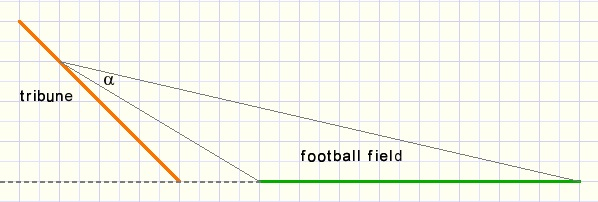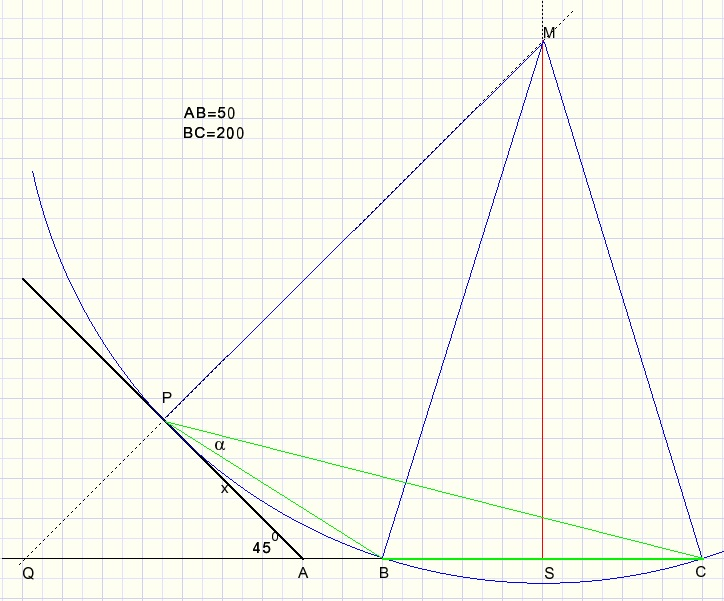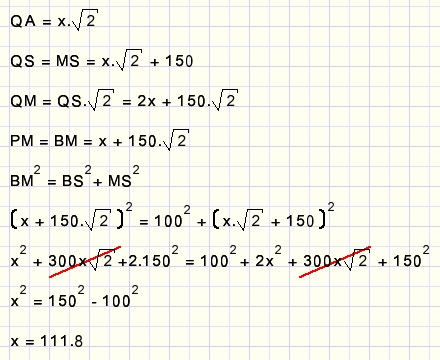The best seat on a tribuneThis geometry problem was presented on facebook by Omid Motahed, math teacher in Beijing.

Question is:
Which seat in a football stadium is best?

Please look at the picture below:A visitor looks at the field at angle a
The bigger this angle, the better.

The solution of this geometry problem requires the lemmas of Thales, see below:Football field BC is the chord of a circle.
Thales' lemma states that from any point on a circle we observe a chord under the same angle.
So LBPC is the same for any point P on the circle.
Also LBQC is the same for any point Q on the circle.
The smaller the radius of the circle the bigger the angle under which we observe the football field.
Please look at the picture below:Our problem may now be redefined as:
find the smallest circle through points B,C,P
This circle will have AP as tangent.

Given are:
AB = 50
BC = 200
Calculation of x = AP:Calculation of a

Thales again:
(a chord is viewed from the circle center under twice the angle as from the circle perimeter)
a = LBMS
MS = 308.11
BS = 100
a = arctan(BS/MS) = 17.980